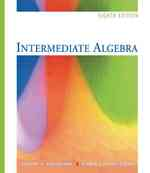### Intermediate Algebra （8 PCK）

• ただいまウェブストアではご注文を受け付けておりません。 ⇒古書を探す
• 製本 Hardcover:ハードカバー版／ページ数 679 p.
• 言語 ENG,ENG
• 商品コード 9780495105527
• DDC分類 512.9

### Table of Contents

`    Basic Concepts and Properties                  1  (43)      Sets, Real Numbers, and Numerical            2  (9)      Expressions      Operations with Real Numbers                 11 (11)      Properties of Real Numbers and the Use of    22 (8)      Exponents      Algebraic Expressions                        30 (14)        Summary                                    40 (1)        Review Problem Set                         41 (2)        Test                                       43 (1)    Equations, Inequalities, and Problem Solving   44 (64)      Solving First-Degree Equations               45 (8)      Equations Involving Fractional Forms         53 (8)      Equations Involving Decimals and Problem     61 (8)      Solving      Formulas                                     69 (11)      Inequalities                                 80 (7)      More on Inequalities and Problem Solving     87 (9)      Equations and Inequalities Involving         96 (12)      Absolute Value        Summary                                    103(1)        Review Problem Set                         104(3)        Test                                       107(1)    Polynomials                                    108(57)      Polynomials: Sums and Differences            109(6)      Products and Quotients of Monomials          115(7)      Multiplying Polynomials                      122(7)      Factoring: Use of the Distributive           129(8)      Property      Factoring: Difference of Two Squares and     137(6)      Sum or Difference of Two Cubes      Factoring Trinomials                         143(8)      Equations and Problem Solving                151(14)        Summary                                    159(1)        Review Problem Set                         160(2)        Test                                       162(1)        Cumulative Review Problem Set (Chapters    163(2)        1--3)    Rational Expressions                           165(59)      Simplifying Rational Expressions             166(6)      Multiplying and Dividing Rational            172(5)      Expressions      Adding and Subtracting Rational              177(8)      Expressions      More on Rational Expressions and Complex     185(10)      Fractions      Dividing Polynomials                         195(6)      Fractional Equations                         201(8)      More Fractional Equations and Applications   209(15)        Summary                                    220(1)        Review Problem Set                         221(2)        Test                                       223(1)    Exponents and Radicals                         224(54)      Using Integers as Exponents                  225(7)      Roots and Radicals                           232(12)      Combining Radicals and Simplifying           244(6)      Radicals That Contain Variables      Products and Quotients Involving Radicals    250(6)      Equations Involving Radicals                 256(5)      Merging Exponents and Roots                  261(7)      Scientific Notation                          268(10)        Summary                                    274(1)        Review Problem Set                         275(2)        Test                                       277(1)    Quadratic Equations and Inequalities           278(55)      Complex Numbers                              279(8)      Quadratic Equations                          287(8)      Completing the Square                        295(5)      Quadratic Formula                            300(8)      More Quadratic Equations and Applications    308(12)      Quadratic and Other Nonlinear Inequalities   320(13)        Summary                                    327(1)        Review Problem Set                         328(2)        Test                                       330(1)        Cumulative Review Problem Set (Chapters    331(2)        1--6)    Linear Equations and Inequalities in Two       333(58)    Variables      Rectangular Coordinate System and Linear     334(15)      Equations      Graphing Nonlinear Equations                 349(8)      Linear Inequalities in Two Variables         357(5)      Distance and Slope                           362(12)      Determining the Equation of a Line           374(17)        Summary                                    387(1)        Review Problem Set                         388(2)        Test                                       390(1)    Conic Sections                                 391(37)      Graphing Parabolas                           392(10)      More Parabolas and Some Circles              402(8)      Graphing Ellipses                            410(5)      Graphing Hyperbolas                          415(13)        Summary                                    425(1)        Review Problem Set                         425(2)        Test                                       427(1)    Functions                                      428(58)      Relations and Functions                      429(7)      Functions: Their Graphs and Applications     436(14)      Graphing Made Easy Via Transformations       450(9)      Composition of Functions                     459(6)      Inverse Functions                            465(9)      Direct and Inverse Variations                474(12)        Summary                                    482(1)        Review Problem Set                         483(2)        Test                                       485(1)    Systems of Equations                           486(64)      Systems of Two Linear Equations in Two       487(8)      Variables      Elimination-by-Addition Method               495(10)      Systems of Three Linear Equations in         505(9)      Three Variables      Matrix Approach to Solving Systems           514(6)      Determinants                                 520(5)      3 X 3 Determinants and Systems of Three      525(7)      Linear Equations in Three Variables      Systems Involving Nonlinear Equations and    532(18)      Systems of Inequalities        Summary                                    541(1)        Review Problem Set                         542(2)        Test                                       544(2)        Cumulative Review Problem Set (Chapters    546(4)        1--10)    Exponential and Logarithmic Functions          550(47)      Exponents and Exponential Functions          551(7)      Applications of Exponential Functions        558(10)      Logarithms                                   568(10)      Logarithmic Functions                        578(7)      Exponential Equations, Logarithmic           585(12)      Equations, and Problem Solving        Summary                                    594(1)        Review Problem Set                         595(1)        Test                                       596(1)    Sequences and Binomial Expansions              597(32)      Arithmetic Sequences                         598(5)      Sums of Arithmetic Sequences                 603(5)      Geometric Sequences                          608(7)      Infinite Geometric Sequences                 615(5)      Binomial Expansions                          620(9)        Summary                                    625(1)        Review Problem Set                         625(2)        Test                                       627(2)Appendix A: Prime Numbers and Operations with      629(12)FractionsAnswers to Odd-Numbered Problems and All           641Chapter Review, Chapter Test, CumulativeReview, and Appendix A ProblemsIndex                                              1`